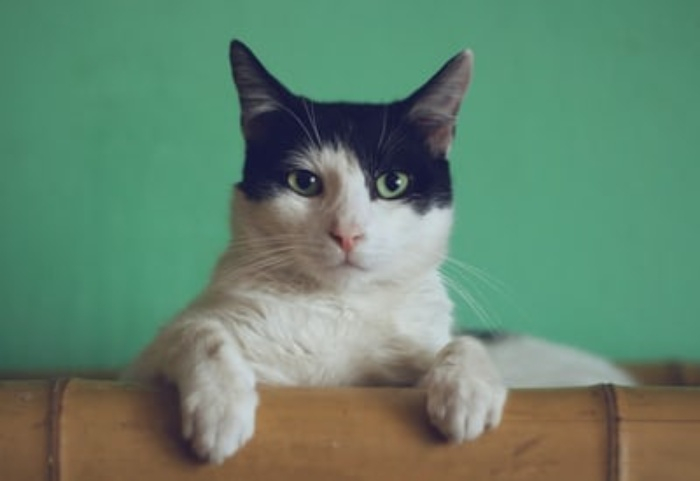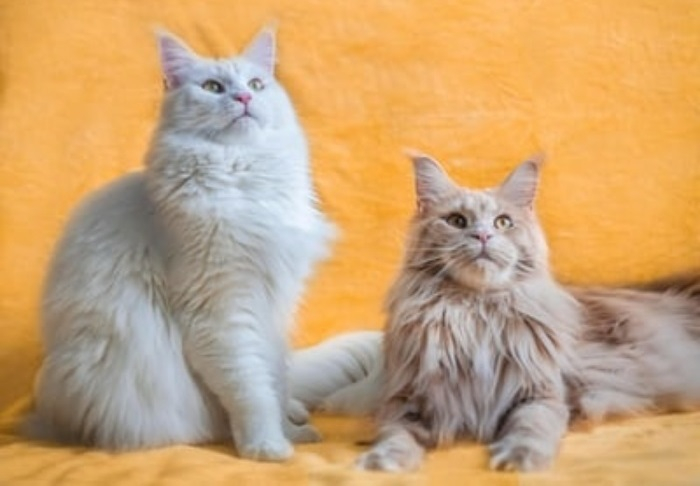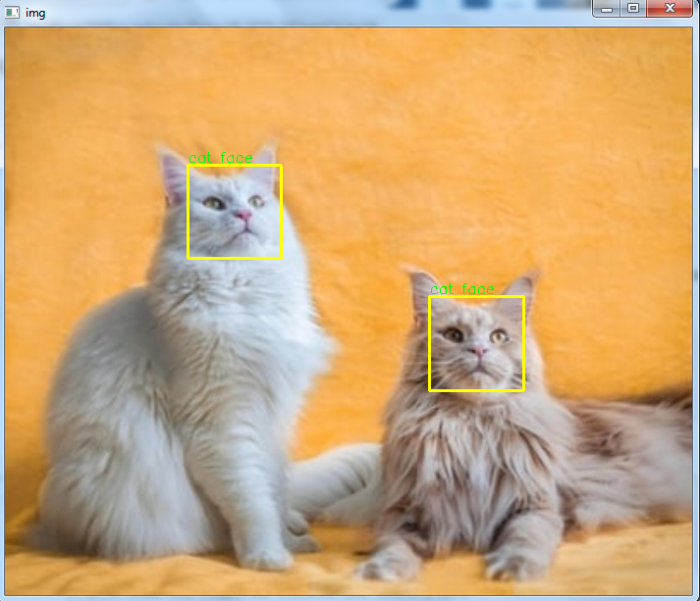# How to detect cat faces in an image in OpenCV using Python?

A haar cascade classifier is an effective object detection method. It is a machine learning based approach. To train a haar cascade classifier for cat face detection, the algorithm initially needs a lot of positive images (images with cat faces) and negative images (images without cat faces). The classifier is trained from these positive and negative images. It is then used to detect cat faces in other images.

We can use already trained haar cascades for smile detection. For smile detection in the input image we need two haar cascades one for face detection and other for smile detection. We will use haarcascade_frontalcatface.xml for cat face detection in the image.

To download the haar cascade for cat face detection click on the haarcascade_frontalcatface.xml file. Open it in raw format, right click and save.

## Steps

To detect cat faces in an image and draw bounding boxes around them, you can follow the steps given below −

• Import the required library. In all the following examples, the required Python library is OpenCV. Make sure you have already installed it.

• Read the input image using cv2.imread(). Specify the full image path. Convert the input image to grayscale.

• Initiate a Haar cascade classifier object cat_cascade = cv2.CascadeClassifier() for cat face detection. Pass the full path of the haar cascade xml file. You can use the haar cascade file haarcascade_frontalcatface.xml to detect cat faces in the image.

• Detect cat faces in the input image using cat_cascade.detectMultiScale(). It returns the coordinates of detected cat faces in (x,y,w,h) format.

• Draw the bounding rectangles around the detected cat faces in the original image using cv2.rectangle().

• Display the image with the drawn bounding rectangles around the cat faces.

Let's have a look at some examples to detect cat faces in the image.

## Example

In this example, we detect cat faces in the input image using a haar cascade.

# import required libraries
import cv2

# convert the input image to grayscale
gray = cv2.cvtColor(img, cv2.COLOR_BGR2GRAY)

# Detects cat faces in the input image
print('Number of detected cat faces:', len(faces))

# if atleast one cat face id detected
if len(faces) > 0:
print("Cat face detected")
for (x,y,w,h) in faces:

# To draw a rectangle in a face
cv2.rectangle(img,(x,y),(x+w,y+h),(0,255,255),2)
cv2.putText(img, 'cat face', (x, y-3),
cv2.FONT_HERSHEY_SIMPLEX, 0.5, (0,255,0), 1)
else:
print("No cat face detected")

# Display an image in a window
cv2.imshow('Cat Image',img)
cv2.waitKey(0)
cv2.destroyAllWindows()


We will use this image as the Input File for this program −## Output

When you execute the program, it will produce the following output −

Number of detected cat faces: 1
Cat face detected


And we get the following output window −## Example

In this example, we detect cat faces in the input image using a haar cascade.

# import required libraries
import cv2

# convert the input image to grayscale
gray = cv2.cvtColor(img, cv2.COLOR_BGR2GRAY)

# Detects cat faces in the input image
print('Number of detected cat faces:', len(faces))

# if atleast one cat face id detected
if len(faces) > 0:
for (x,y,w,h) in faces:
print("Cat face detected")

# To draw a rectangle in a face
cv2.rectangle(img,(x,y),(x+w,y+h),(0,255,255),2)
cv2.putText(img, 'cat face', (x, y-3),
cv2.FONT_HERSHEY_SIMPLEX, 0.5, (0,255,0), 1)
else:
print("No cat face detected")

# Display an image in a window
cv2.imshow('Cat Image',img)
cv2.waitKey(0)
cv2.destroyAllWindows()


We will use this image as the Input File for this program −On execution, it will produce the following output

Number of detected cat faces: 2
Cat face detected
Cat face detected


And we get the following output window −